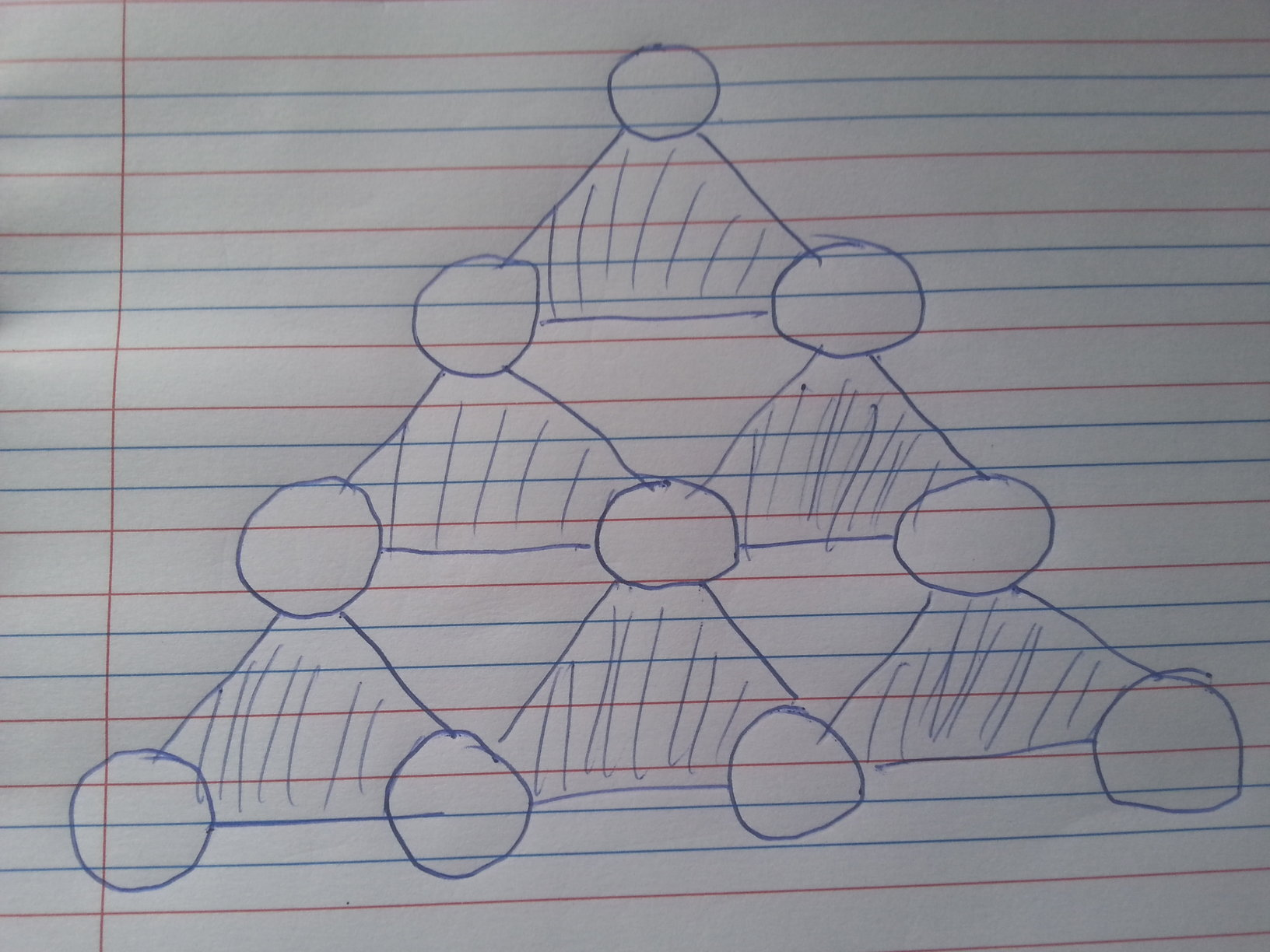# The Mystic Triangle

Logic Level 5Insert the digits 0 to 9 without repetition in the 10 circles at the vertices of the figure above such that the sum of the numbers in the vertices of each shaded triangle is equal.

Among the solutions that exist, the vertex in the Center circle can have only certain possible values. Find the product of those possible values.

×